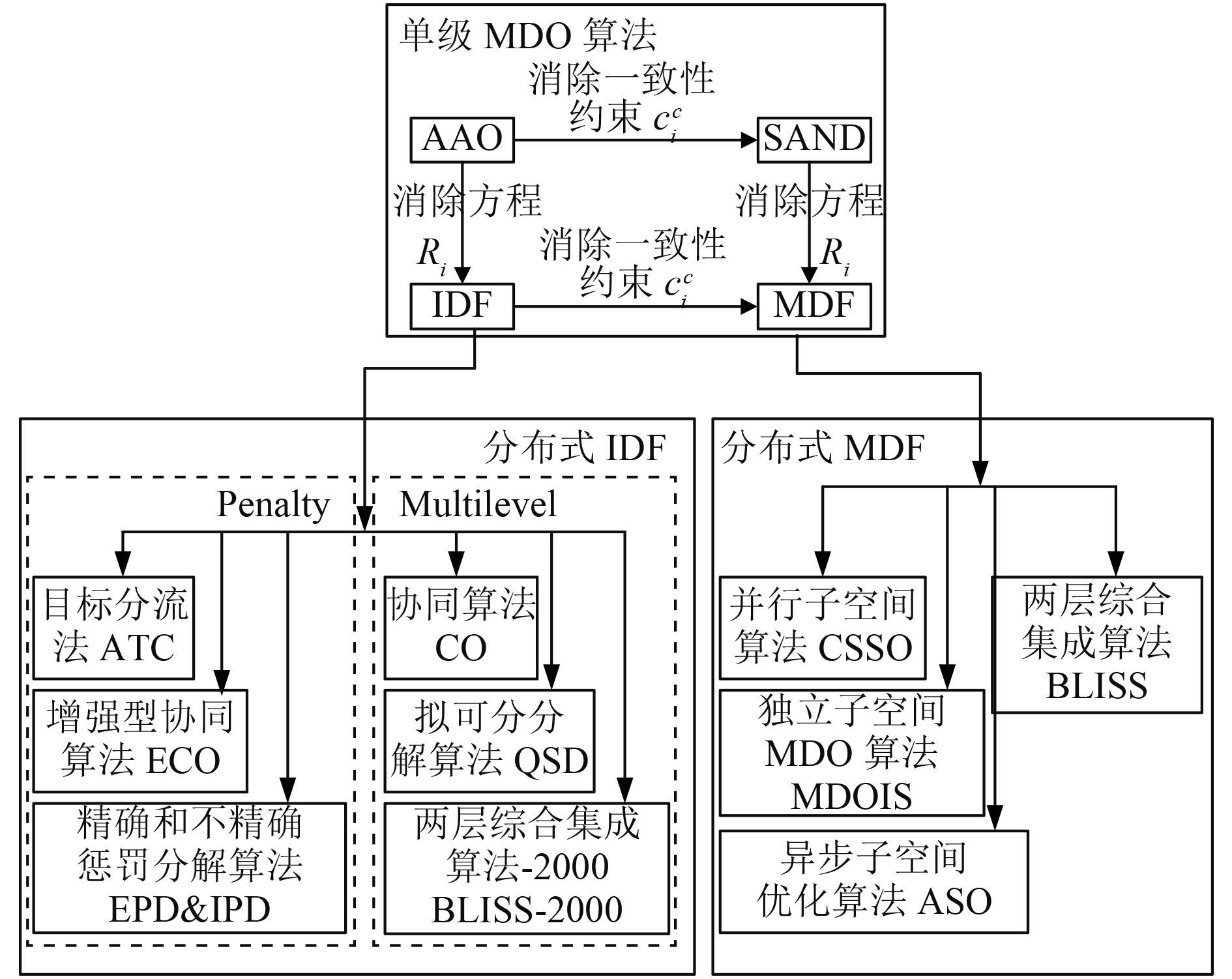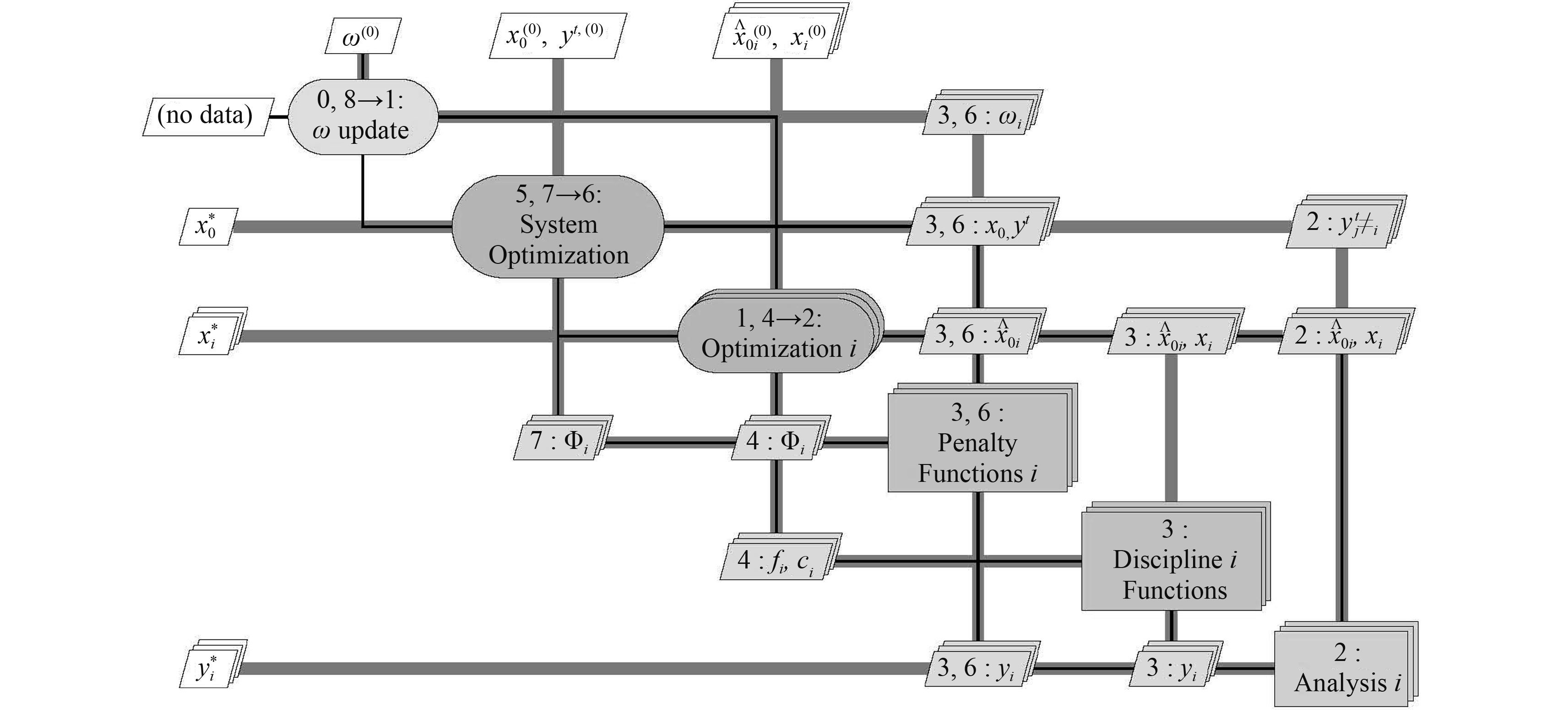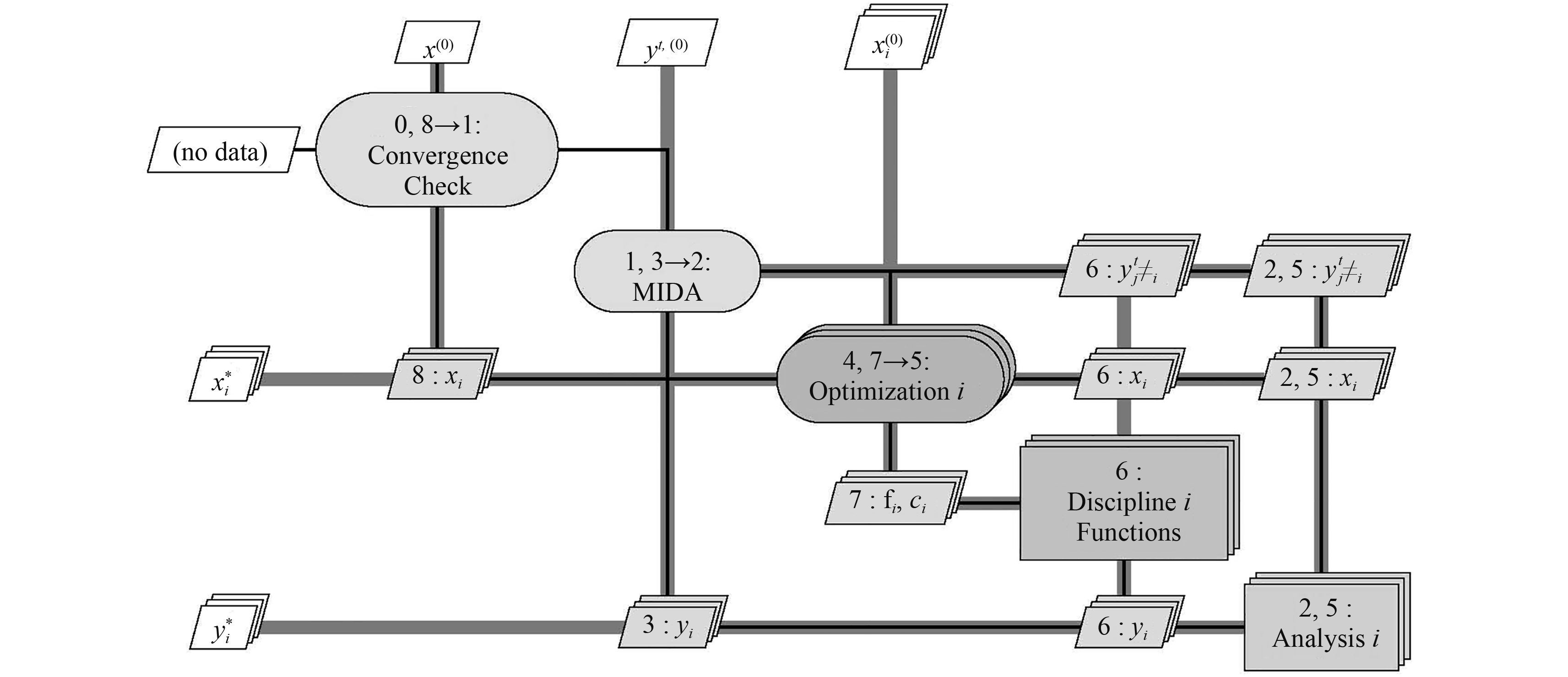﻿ 多学科设计优化算法研究综述
 舰船科学技术2017, Vol. 39Issue (2): 1-5,47PDF

Review of the multidisciplinary design optimization algorithm
YANG Lei, WEI Xi-zhong, ZHAO Feng, LI Sheng-zhong
China Ship Scientific Research Center, Wuxi 214082, China
Abstract: With the development of the Multidisciplinary Design Optimization (MDO) technology, it will become the general trend of the optimization in the future. For a better use of MDO to solve ship design problem, this paper provided a survey and classification of the main MDO algorithms that have been present in recent literatures. A brief introduction was carried out for some developed MDO algorithms, but a more details of the application environment as well as performance features were present here for several developing ones. Finally, a discussion on the drawback and develop trend of MDO algorithm were performed, and several suggestions were given.
Key words: MDO     MDO algorithm     MDO algorithm classification
0 引 言

MDO 从理论层面解决了多系统的协同设计问题，MDO 算法则是这一理论的具体实现方法。显然，由于设计问题的不同，所要求的实现方法也就不同，从而发展了各种各具特点的 MDO 算法。这就要求设计研究人员对各不同 MDO 算法有一定的了解，为此，本文根据相关文献，对近年来发展的 MDO 算法进行总结，并重点介绍几种特殊 MDO 算法应用目的、分解方法及性能特点，为实际应用提供参考。最后，讨论了当前 MDO 算法研究中存在的问题，并提出了若干建议。

1 MDO 算法概述

MDO 算法又称为 MDO 过程、MDO 体系结构（MDO Architecture），区别于传统最优化方法，MDO 算法关注优化过程的组织方式、耦合信息的传递和协同策略，而不是搜索策略本身。为便于阐述 MDO 算法原理及不同 MDO 算法的特点，首先给出 MDO 算法的一般数学表达形式，如式（1）所示，该式又被称为同时优化算法（All-at-once，AAO）

 $\begin{array}{l} {\rm{Find: }}{\left[ {x,{y^t},y,\bar y} \right]^{{\rm{ }}{\rm T}}}{\rm{ }} \text{，}\\ {\rm{Min: }}{f_0}(x,y) + \sum\limits_i {{f_i}({x_0},{x_i},{y_i})}\text{，} \\ {\rm{ S}}{\rm{. t}}{\rm{.: }}{c_0}(x,y) \geqslant 0\text{，} \\ \;\;\;\;\;\;\;\;\;\;{c_i}(({x_0},{x_i},{y_i}) \geqslant 0\;\;\;\;\;\;\;\;\;\;\;\;\;\;\;\;\;\;i = 1, \cdots ,N\text{；}\\ \;\;\;\;\;\;\;\;\;\;c_i^c = y_i^t - {y_i} = 0\;\;\;\;\;\;\;\;\;\;\;\;\;\;\;\;\;\;\;i = 1, \cdots ,N\text{；}\\ \;\;\;\;\;\;\;\;\;\;{R_i}({x_0},{x_i},y_{j \ne i}^t,{{\bar y}_i},{y_i}) = 0\;\;\;\;\;\;i = 1, \cdots ,N\text{。} \end{array}$ (1)

AAO 算法对所有变量，包括设计变量、状态变量、输入/输出耦合变量，进行同时优化，问题规模大、收敛速度慢，实际应用少，但是，通过对约束条件的不同处理可得到更高效的 MDO 算法。图 1 MDO 算法分类 Fig. 1 Classification of the MDO Architecture
2 多级分布式 MDO 算法 2.1 几种典型多级分布式 MDO 算法

1）并行子空间算法（Concurrent Subspace Optimization，CSSO）[11-12]是最早提出的多级分布式 MDO 算法之一。其基本思想是将系统优化问题分解为若干个设计变量不相交的子优化问题，各子系统优化的目标函数均为原系统优化问题的目标函数，各子学科只对本学科局部设计变量进行优化，所需的耦合输入变量用近似模型代替。CSSO 需要进行复杂的多学科分析（Multidisciplinary Analysis，MDA），系统分解要求各子学科设计变量集不相交，在工程上较难满足，限制了该方法的实际应用。

2）协同优化算法（Collaborative Optimization，CO）是目前研究最多、应用研究最为广泛的 MDO 算法。其基本思想是将系统进行层次分解，各子系统只与父系统进行数据交换，系统层目标函数为原问题目标函数，各子系统以最小化与系统分配的目标方案的差异为目标函数，系统层通过一致性约束协调各子学科进行并行优化。CO 算法，子学科优化自治性高，获得工程设计师的青睐，但其系统优化问题不满足 KKT 最优化条件，其数学收敛性尚未得到证明[14-15]

3）增强型协同算法（Enhanced Collaborative Optimization，ECO）的基本思想是将 CO 算法的系统层与子系统层目标函数对调，增强各子学科对目标函数的“影响力”。研究表明，ECO 比 CO 的分析次数更少，但构建及更新子学科模型需要增加额外的时间。

4）两层综合集成算法（Bilevel Integrated System Synthesis，BLISS）将设计变量分为全局（系统）设计变量和学科局部设计变量，应用泰勒展开式构造原问题目标函数近似式，利用梯度指导的方法，交替优化全局设计变量和学科局部设计变量，直到获得系统最优解。这一过程需进行大量的偏导数计算，优化计算成本高，通过采用响应面方法、Kring 方法可得到缓解。

5）目标分流法（Analytical Target Cascading，ATC）的基本思想是将系统设计要求，包括目标函数、约束条件，按照层次系统由上到下逐级传递到子系统，各子系统围绕系统设计要求对各学科局部设计变量进行优化，直到获得满足目标的设计方案，若无法完全满足目标值，则返回最接近目标的设计方案，ATC 与 CO 算法思想相似。目前，关于ATC算法的性能研究，目前尚不多，但已在汽车、桥梁、飞机、船舶等领域开展应用研究。

2.2 几种特殊的多级分布式 MDO 算法 2.2.1 精确和非精确惩罚分解算法

 \begin{array}{l} {\rm{Find}}:[{{\hat x}_{0i}},{x_i}]\text{，}\\ {\begin{aligned} {\rm{Min}}: & {f_i}({{\hat x}_{0i}},{x_i},{y_i}({{\hat x}_{0i}},{x_i},y_{j \ne i}^t)) +\\ & {\Phi _i}({{\hat x}_{0i}} - {x_0},y_i^t - {y_i}({{\hat x}_{0i}},{x_i},y))\text{，} \end{aligned}} \\ {\rm{S}}{\rm{.t}}{\rm{.}}:{\rm{ }}{c_i}({{\hat x}_{0i}},{x_i},{y_i}({x_{0i}},{x_i},y_{j \ne i}^t)) \geqslant 0\text{。} \end{array} (2)

EPD 和 IPD 的系统优化问题相同，它是以最小化各子系统惩罚函数Φi 之和为目标的无约束优化问题，如下式所示：

 $\begin{split} \\[-10pt] {\rm{Find}}: & [{x_0},{y^t}]\text{，}\\ {\rm{Min}}: & \sum\limits_{i = 1}^N {{\omega _i}\Phi _i^*\left( {{x_0},{y^t}} \right)} = \\ & \sum\limits_{i = 1}^N {{\omega _i}{\Phi _i}\left( {{{\hat x}_{0i}} - {x_0},y_i^t - {y_i}\left( {{{\hat x}_{0i}},{x_i},y_{j \ne i}^t} \right)} \right)} \text{。} \end{split}$ (3)图 2 EPD&IPD算法结构图 Fig. 2 Diagram of the EPD&IPD Architecture
2.2.2 独立子空间 MDO 算法

 $\begin{array}{l} {\rm{Find:}}[{x_i}]\text{，}\\ {\rm{Min: }}{f_i}\left( {{x_i},{y_i}\left( {{x_i},y_{j \ne i}^t} \right)} \right)\text{，}\\ {\rm{ S}}{\rm{.t}}{\rm{. : }}{c_i}\left( {{x_i},{y_i}\left( {{x_i},y_{j \ne i}^t} \right)} \right) \geqslant 0\text{。} \end{array}$ (4)

MDOIS 的最大特点是，不存在系统优化问题。如图 3 所示，它是通过 MDA 过程来引导各学科设计优化过程，当 MDA 过程收敛时，即获得系统最优解，它属于分布式 MDF 算法。研究表明，在满足假定条件的前提下，MDOIS 均能获得最优解；基准试验研究发现，在很多数情况下，获得同样的最优解，MDOIS 比 MDF 过程所需的分析次数还要少，但在应用的灵活性上却不及后者图 3 MDOIS 算法结构图 Fig. 3 Diagram of the MDOIS Architecture
2.2.3 异步子空间法

 $\begin{array}{l} {\rm{Find}}:[{x_0},{x_k}]\text{，}\\ {\rm{Min}}:{f_0}(x,y(x,y)){\rm{ }} + \sum\limits_k {{f_k}({x_0},{x_k},{y_k}({x_0},{x_k},{y_{j \ne k}}))} \text{，}\\ {\rm{S}}{\rm{.t}}{\rm{.}}:{c_0}(x,y(x,y)) \geqslant 0\text{，}\\ {\rm{ }}{c_k}({x_0},{x_k},{y_k}({x_0},{x_k},{y_{j \ne k}})) \geqslant 0{\rm{ for all }}k\text{。} \end{array}$ (5)
 $\begin{array}{l} {\rm{Find}}:\left[ {{x_i}} \right]\text{，}\\ {\rm{Min}}:{f_0}(x,y(x,y)) + {f_i}({x_0},{x_i},{y_i}({x_0},{x_i},{y_{j \ne i}}))\text{，}\\ {\rm{S}}{\rm{.t}}{\rm{.}}:{c_i}({x_0},{x_i},{y_i}({x_0},{x_i},{y_{j \ne i}})) \geqslant 0{\rm{ }}\text{。} \end{array}$ (6)

ASO 算法属于分布式 MDF 算法，有复杂的 MDA 过程，相应的，需要进行系统灵敏度计算，可应用耦合优化后灵敏度方程来计算，也可应用耦合伴随方程计算。飞机机翼的 MDO 应用研究表明，应用 ASO 算法不仅大幅减少了空气动力学分析计算的次数，甚至一定程度上还减少了结构分析计算的次数；2 个学科分析计算耗时差距越大，总的分析计算次数减少越明显，ASO 过程的分析次数也越接近 MDF 过程。也即是说，只有当 2 个学科分析计算时间相差很大时，ASO 算法才具有优势。

3 结 语

MDO 算法研究已经取得了丰硕的成果，为工程应用提供了更多的选择，但同时又带来新的挑战：即面对给定的 MDO 问题，选择哪一种 MDO 算法，建模更快捷、优化效率更高、优化质量更好，也就是说，需要了解不同 MDO 算法对于特定问题的解决能力。然而，由于不同研究人员实现同一算法的方式、计算环境、测试算例存在差异，特别是研究人员对算法的熟悉程度或偏好不同，都容易引起评估结果出现偏差，甚至相互矛盾。为客观起见，应尽可能在一套规范的方法和流程下，对不同 MDO 算法进行对比研究，从而准确把握不同 MDO 算法的应用要求和性能特点，这类似于船舶水动力性能试验研究中的标模基准试验研究，本文称之为“MDO 算法基准试验研究”，国内外在该领域已开展了一些研究[28-32]，但在以下 2 个方面还需做进一步的深入研究：

1）基准测试算例简单，不利于评估 MDO 算法在处理大规模工程问题时的性能。目前发展的基准测试算例大多为简化后的工程设计模型，维度低、分析模型简单、计算复杂度低，而实际工程系统设计变量、约束模型可能达到 103 量级，一次学科分析可能需要花费几小时甚至几十小时，这些分析计算还可能是由不同的计算机采用不同的程序或软件完成，而 MDO 算法表现的性能又与问题规模密切相关。因此，应进一步开展具有大工程系统特征的基准测试算例研究。

2）MDO 基准试验研究针对的对象过于集中，不利于新算法的推广应用。从发表的文献来看，目前的 MDO 基准试验比较研究大多是针对 MDF，IDF，CO，CSSO 等少数几种发展时间较长、已较成熟的算法，而对 ASO，MDOIS 等新算法的对比研究凤毛麟角，不利于新算法的推广应用。

  ﻿SOBIESZCZANSKI-SOBIESKI.J. A linear decomposition method for large optimization problems-blueprint for development[R]. NASA Technical Memorandum 83248, Feb. 1982.  GIESING HOSEPH P, BARTHELEMY J M. A summary of industry MDO application and needs[C]//An AIAA White Paper, 7th AIAA/USAF/NASA/ISSMO Symposium on Multidisciplinary Analysis and Optimization, 1998.  OLDS, JOHN R. Multidisciplinary design techniques applied to conceptual aerospace vehicle desig[D]. North Carolina State University, 1993.  GIUNTA A A, GOLIVIDOV O, KNILL D L, et al. Multidisciplinry design optimization of advanced aircraft configurations[R]. MAD Center Report 96-06-01.  JOEL M G. Multidisciplinary design optimization of a strut-braced wing aircraft[M]. Virginia Polytechnic Institute and State University, April 13, 1998.  蔡伟. 不确定性多目标MDO理论及其在飞行器总体设计中的应用研究[D]. 长沙:国防科学技术大学, 2008. http://cdmd.cnki.com.cn/Article/CDMD-90002-2009213878.htm  SRINIVAS K, SOBIESZCZANSKI-SOBIESKI J. Multidisciplinary design optimization-some formal methods, framework requirement, and application to vehicle design[C]//Int.J.VehicleDesign(Special Issue), 3-22.  CRAMER E J, DENNIS Jr J E, FRANK P D, et al. Problem formulation for multidisciplinary optimization[J]. SIAM Journal on Optimization, 1994, 4 (4): 754–776. DOI: 10.1137/0804044  HAFTKA R T. Simultaneous analysis and design[J]. AIAA Journal, 1985, 7 (7): 1099–1103.  BALLING R J, SOBIESZCZANSKI-SOBIESKI J. Optimization of coupled systems:a critical overview of approaches[J]. AIAA Journal, 1996, 34 (1): 6–17. DOI: 10.2514/3.13015  SELLAR R S, BATILL S M, RENAUD J E. Response surface based, concurrent subspace optimization for multidisciplinary system design[C]//34th AIAA Aerospace Sciences and Meeting Exhibit, 1996.  RENAUD, JOHN E. Sequential approximation in non-hierarchic system decomposition and optimization:a multidisciplinary design tool[D]. Rensselaer Polytechnic Institute, 1992.  KROO J, ALTUS S, SOBIESZCZANSKI-SOBIESKI J, et al. Multidisciplinary optimization methods for aircraft preliminary design[J], AIAA-94-4325, 1994.  ALEXANDROV N M, LEWIS R M. Analytical and computational aspects of collaborative optimization for multidisciplinary design[J]. AIAA Journal, 2002, 40 (2): 301–309. DOI: 10.2514/2.1646  DEMIGUEL A V, MURRAY W. An analysis of collaborative optimization methods[C]//8th AIAA/USAF/NASA/ISSMO Symposium on Multidisciplinary Analysis &Optimization, Long Beach, CA, 2000.  BRIAN R, ILANKROO. Enhanced collaborative optimization:application to an analytic test problem and aircraft design[C]//In 12th AIAA/ISSMO Multidisciplinary Analysis and Optimization Conference, number September, Victoria, British Columbia, Canada, September 2008.  BRIAN D R. Aircraft family design using enhanced collaborative optimization[D]. Stanford University, 2008.  JAROSLAW, SOBESZCZANSKI-SOBIESKI. Bi-level integrated system synthesis(BLISS)[J]. NASA/TM-1998-208715;  KIM H M, MICHELENA N F, PAPALAMBROS P Y, et al. Target cascading in optimal system design[J]. Journal of Mechanical Design, 2003, 125 (3): 474–480. DOI: 10.1115/1.1582501  ANGEL-VICTOR D. Two decomposition algorithms for nonconvex optimization problems with global variables[D]. Stanford University, 2001.  DEMIGUEL V, MURRAY W. A local convergence analysis of bileveldecomposition algorithms[J]. Optimization and Engineering, 2006, 7 (2): 99–133. DOI: 10.1007/s11081-006-6835-3  MOON-KYUN S, GYUNG-JIN P. Multidisciplinary design optimization based on independent subspaces[J]. International Journal for Numerical Methods in Engineering, 2005 (64): 599–617.  SI Yi, SHIN J K, PARK G J. Comparison of MDO methods with mathematical examples[J]. Structural and Multidisciplinary Optimization, 2008 (39): 391–402.  IAN R .Chittick, Joaquim R. R. A.Martins. An asymmetric suboptimization approach to aerostructural optimization[J]. Optimization and Engineering, 2009, 10 (1): 133–152. DOI: 10.1007/s11081-008-9046-2  MARTINS J R R A, ALONSO J J, REUTHER J J. A coupled-adjoint sensitivity analysis method for high-fidelity aero-structural design[J]. Optimization and Engineering, 2005, 6 (1): 33–62. DOI: 10.1023/B:OPTE.0000048536.47956.62  SRINIVAS KODIYALAM. Evaluation of methods for multidisciplinary design optimization(MDO), PhaseⅠ[C]//NASA/CR-1998-20876.  SRINIVAS KODIYALAM, CHARLES YUAN. Evaluation of methods for multidisciplinary design optimization(MDO), Phase Ⅱ[C]//NASA/CR-2000-210313.  赵敏, 操安喜, 崔维成. 多学科设计优化方法的比较[J]. 中国造船, 2008, 49 (3): 68–78.  孔凡国, 李钰. 多学科设计优化方法与传统设计优化方法的比较研究[J]. 计算机工程与科学, 2008, 30 (7): 136–138.  HULME K F, BOLEBAUM C L. A comparison of solution strategies for simulation-based multidisciplinary design optimization[C]//AIAA-98-4977;  龚春林, 谷良贤, 袁建平. 基于全局优化算法的多学科优化计算构架[J]. 西北工业大学学报, 2009, 27 (1): 52–56.  李世海. 多学科设计优化(MDO)算法研究[D]. 中国地质大学(北京)硕士学位论文, 2009. http://cdmd.cnki.com.cn/Article/CDMD-11415-2009076095.htm  Nathan P. Tedford, Joaquim R.R.A.Martins. Benchmarking multidisciplinary design optimization algorithms[J]. Optim Eng, 2010 (11): 159–183.